﻿ 不同绕组结构的旋转变压器输出电压波形与谐波对比分析

# 不同绕组结构的旋转变压器输出电压波形与谐波对比分析Comparative Analysis of Output Voltage Waveform and Harmonic of Resolvers with Different Winding Structures

Abstract: The accuracy of resolver is one of important indicators. The distribution of windings has a greater influence on the sine of its output voltage waveform, and it is one of the main factors that determine the accuracy. In this paper, a resolver is simulated by finite element analysis software Ansoft Maxwell, to compare and analyze the influence of the double-layer short-distance winding and the concentric sine winding on the sine of the resolver’s output voltage waveform, and to compare and analyze the harmonics. In a more visual and intuitive way to explain which structure of the winding, can improve the accuracy of the rotary transformer, more suitable for the design of the rotary transformer, and provides a certain theoretical basis for the design of the resolver.

1. 引言

2. 电磁仿真计算Table 1. Main parameters of resolver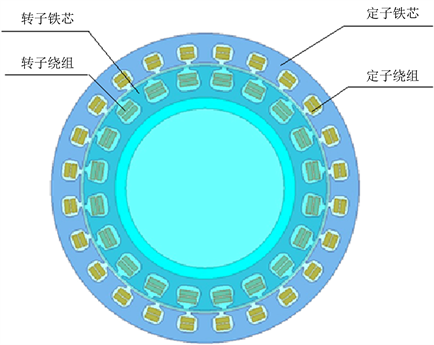Figure 1. Electromagnetic simulation model of resolver

2.1. 双层短距绕组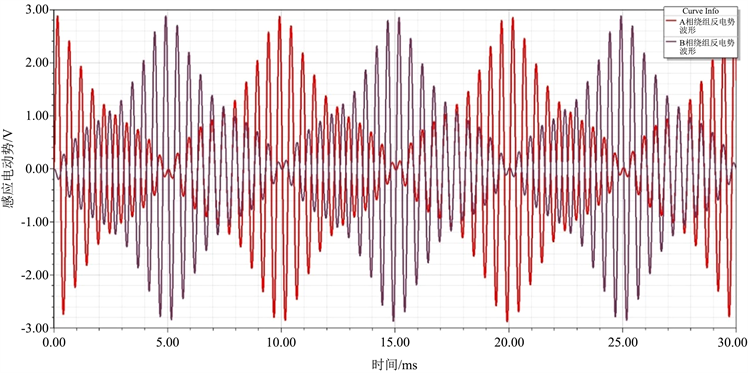Figure 2. Output voltage waveform of double layer short distance winding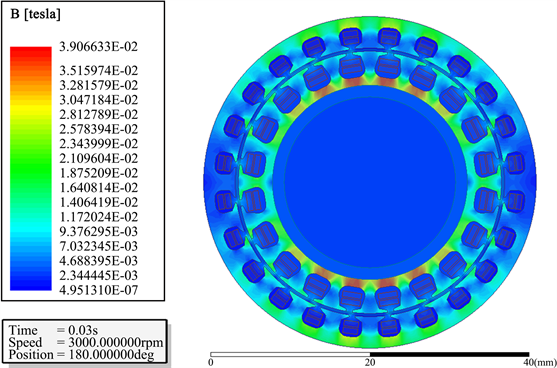Figure 3. Cloud diagram of magnetic field distribution of double-layer short distance winding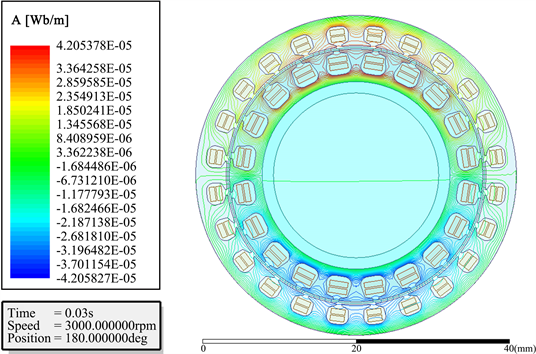Figure 4. Cloud diagram of magnetic field line distribution of double-layer short pitch winding

2.2. 同心式正弦绕组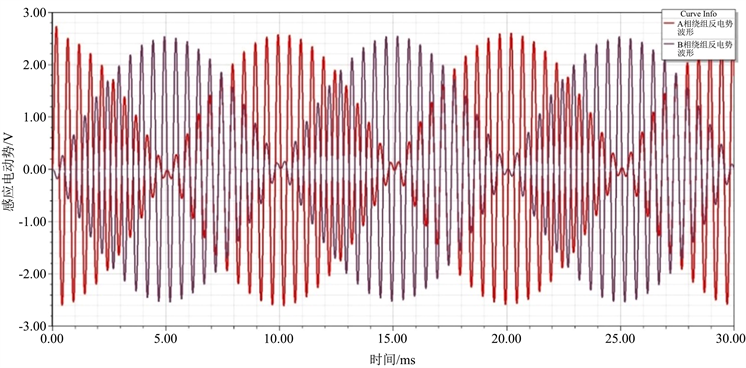Figure 5. Output voltage waveform of sine cosine winding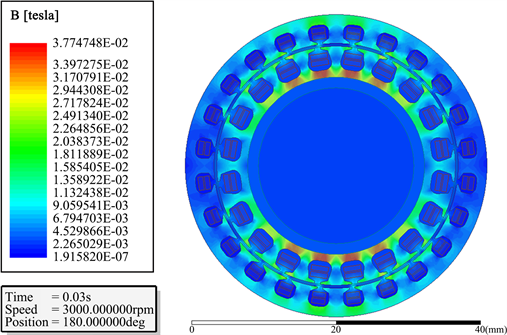Figure 6. Nephogram of magnetic field distribution of sinusoidal winding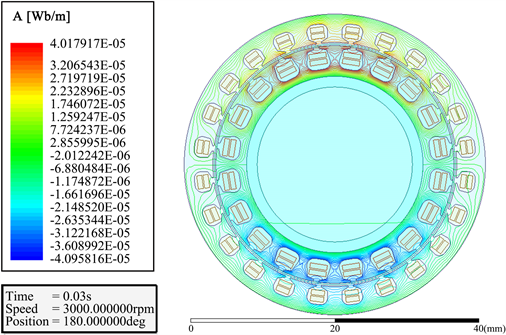Figure 7. Cloud diagram of magnetic field line distribution of sinusoidal winding

3. 谐波分析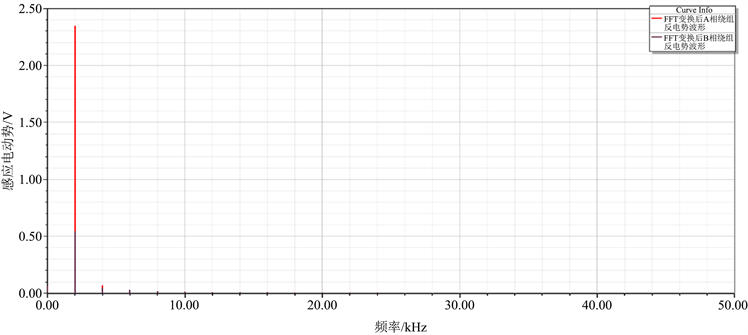Figure 8. Harmonic diagram of double layer short distance winding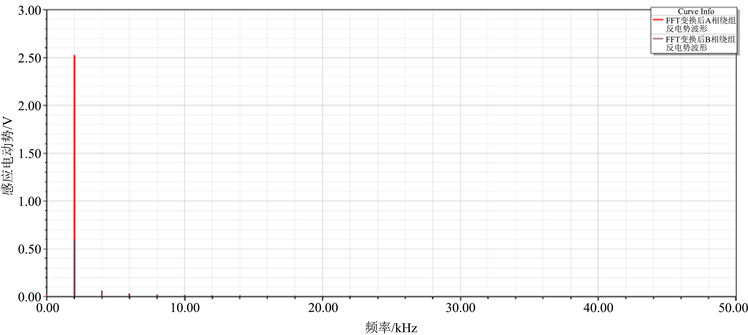Figure 9. Harmonic diagram of concentric sine windingTable 2. Fundamental and harmonic amplitude

4. 结论

 朱青. 无接触旋转变压器[J]. 微电机, 1984(2): 22-27.

 许兴斗, 王永博, 钱俊阳, 周竞捷, 周奇慧. 轴向窜动旋转变压器的优化设计[J]. 微特电机, 2020, 48(9): 31-33+38.

 李立娜, 李大超, 袁永杰. 无刷旋转变压器的磁路设计及仿真分析[J]. 微特电机, 2015, 43(8): 53-56.

 沈训欢, 谷爱昱, 梁国荣. 无接触式旋转变压器的设计及仿真[J]. 防爆电机, 2013, 48(3): 5-9.

 许兴斗, 王永博, 周竞捷, 周奇慧. 一种旋转变压器电气精度的仿真分析方法[J]. 微特电机, 2020, 48(12): 11-13+18.

 西安微电机研究所. 控制微电机设计[M]. 北京: 机械工业出版社, 1979.

 上海微电机研究所. 微特电机[M]. 上海: 上海科技技术出版社, 1983.

 赵博, 张洪亮. Ansoft12在工程电磁场中的应用[M]. 北京: 中国水利水电出版社, 2010.

Top